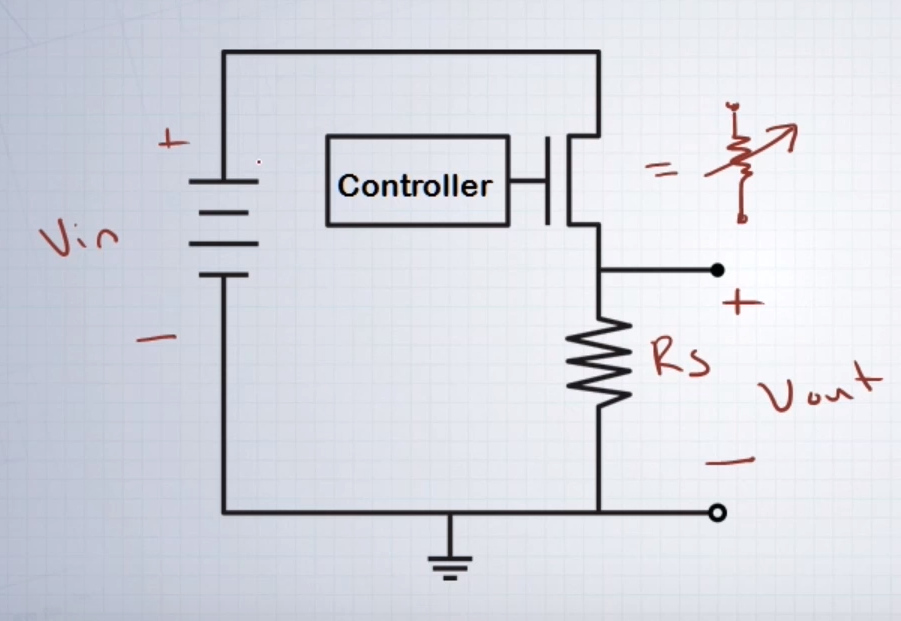# What is a linear switching regulator Circuit - Basics

2 posts / 0 newOffline

Joined: Apr 17, 2018

Replies: 119
What is a linear switching regulator Circuit - Basics
October 24, 2018 - 1:04pm

Hi, I am just getting started with switching circuits. I asked my professor for a very simple switching circuit that I could start learnign and he sugested me "Linear Switching Regulator using MOSFET".

I have already spent more than an hour online trying to understand this but I am getting only Buck and Boost converter. So finally decided to post it up here.

Can someone tell me what is a Linear Switching regulator circuit and the basics for building or simulating one.Offline

Joined: Aug 16, 2016

Replies: 951
Linear Regulator Operation using Transistor
October 24, 2018 - 3:29pm

You can consider Linear switching circuit as a very simple and crude way of stepping down higher voltages to lower level voltages. For example you can use this circuit to converter a 12V battery voltage to 5V for powering you digital electronics like you microcontroller.

To understand Linear Switching circuit we first need to understand how a voltage divider works. You can read more on voltage/Potential divider here. But simply put it has two fixed resistors R1 and R2 using which we can obtain a pre-defined output voltage (Vout) across R2  for fixed value of input voltage (Vin). The formulae to calculate this output voltage is

Vout = (Vin*R2)/(R2+R1)

The same concept is applied in a Linear Switching Circuit, but here the values of R1 and R2 cannot be fixed since we should get a fixed output voltage for all values of input voltage. In other words the output voltage should not be dependent on the input voltage. To tackle this we make one value of Resistor as variable say the resistor R2 is made variable, this way we can increase/decrease the value of R2 to get the required output voltage.

But this change in resistance should happen automatically, so we employ a switching device like transistor or MOSFET in place of R2 . Any switching device can act as a variable resistor when it is operating in  Linear mode(more on that later). The image below should give you a rough idea on the conversion.

To control the resistance offered by the MOSFET we have to control the Gate Source Voltage Vgs. For different value of Vgs you will get the MOSFET operatign in different points at the linear regin. The below graph shows the VI curve for different Vgs

You might have guessed the remaining by now, the Vgs of the of the MOSFET is controlled by controller which has the output voltage value as a feedback. So irrespective of the input voltage the output voltage can be regulated by by controlling the switching of the MOSFET.

These designs are rarely used in circuits today, mainly because of its low efficiency than that of a buck converter. But since the complexity and cost is low people tend to use it in applications where is the output power is low. A basic MOSFET linear regulator is shown below